05 217 385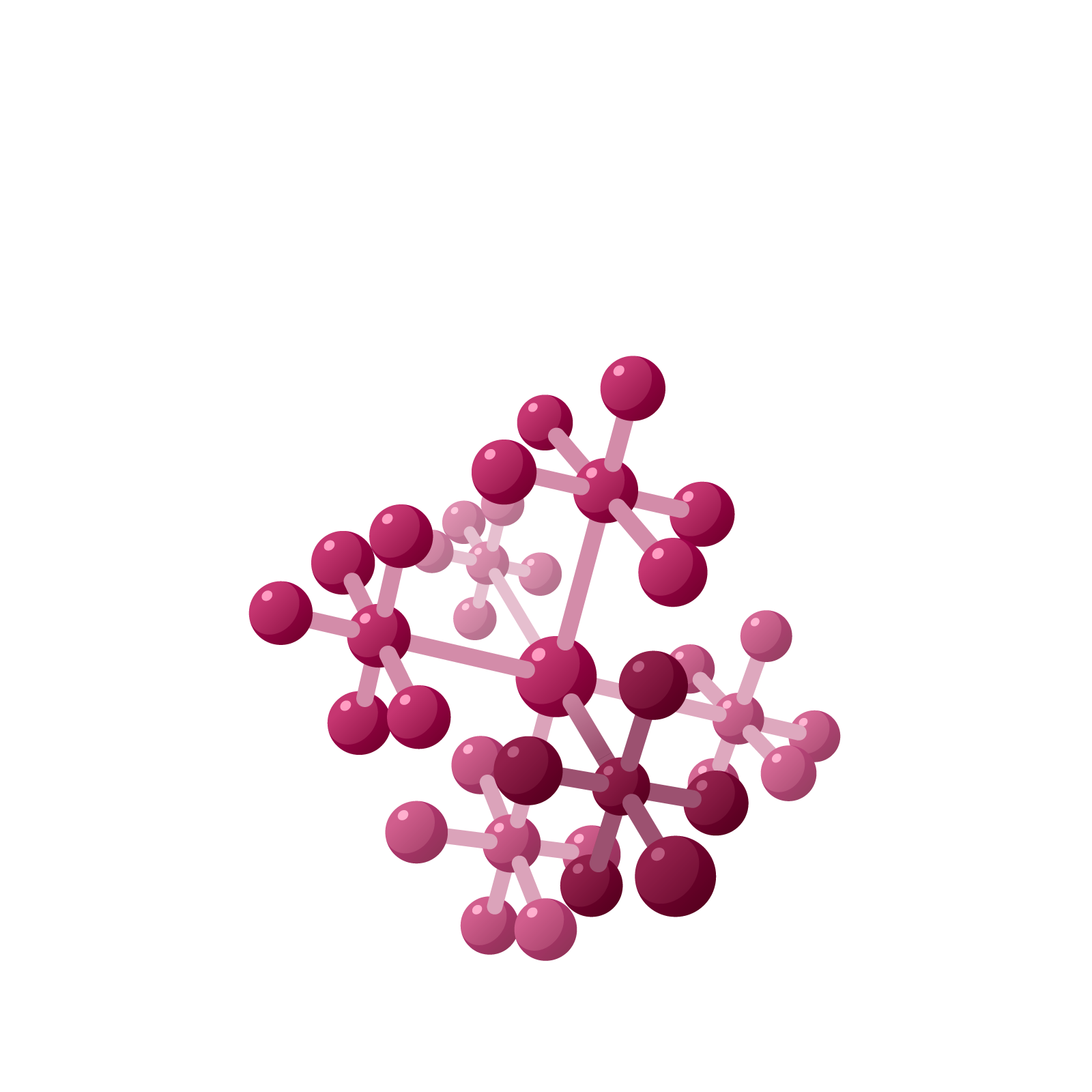# EXPLORATION 1: SQUARES AND SQUARE ROOTS

Napier claimed that his checkerboard is also capable of computing integer square root approximations to numbers. For example, his checkerboard can show that $145=12^2+1$ with $12$ being the integer part of $\sqrt{145}$, and that $1000=31^2+39$ with $31$ being the integer part of $\sqrt{1000}$, and so on.

To get a sense of how one might do this, consider first this picture of $11 \times 11$ to give the square number $121$.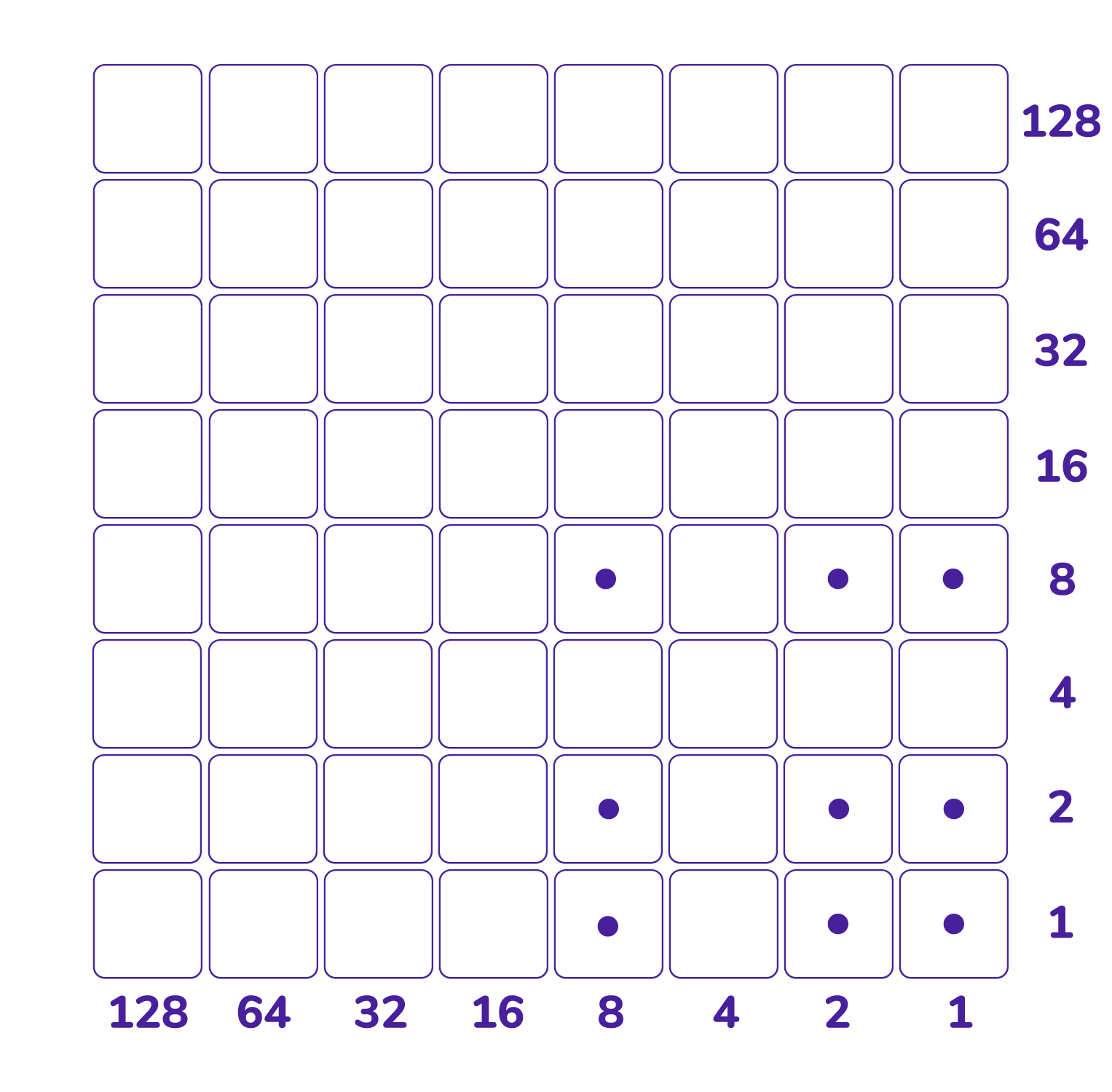Notice the symmetry about the north-west diagonal: the picture has a pattern of dots on the bottom row, the same pattern of dots in the right column, and the same pattern appears on the diagonal too. Also, each dot in the interior of the picture sits above a dot in the bottom row and to the left of a dot in the rightmost column. All pictures of numbers squared will have such symmetry.

Sliding the dots downwards reveals $11 \times 11$ as $121$.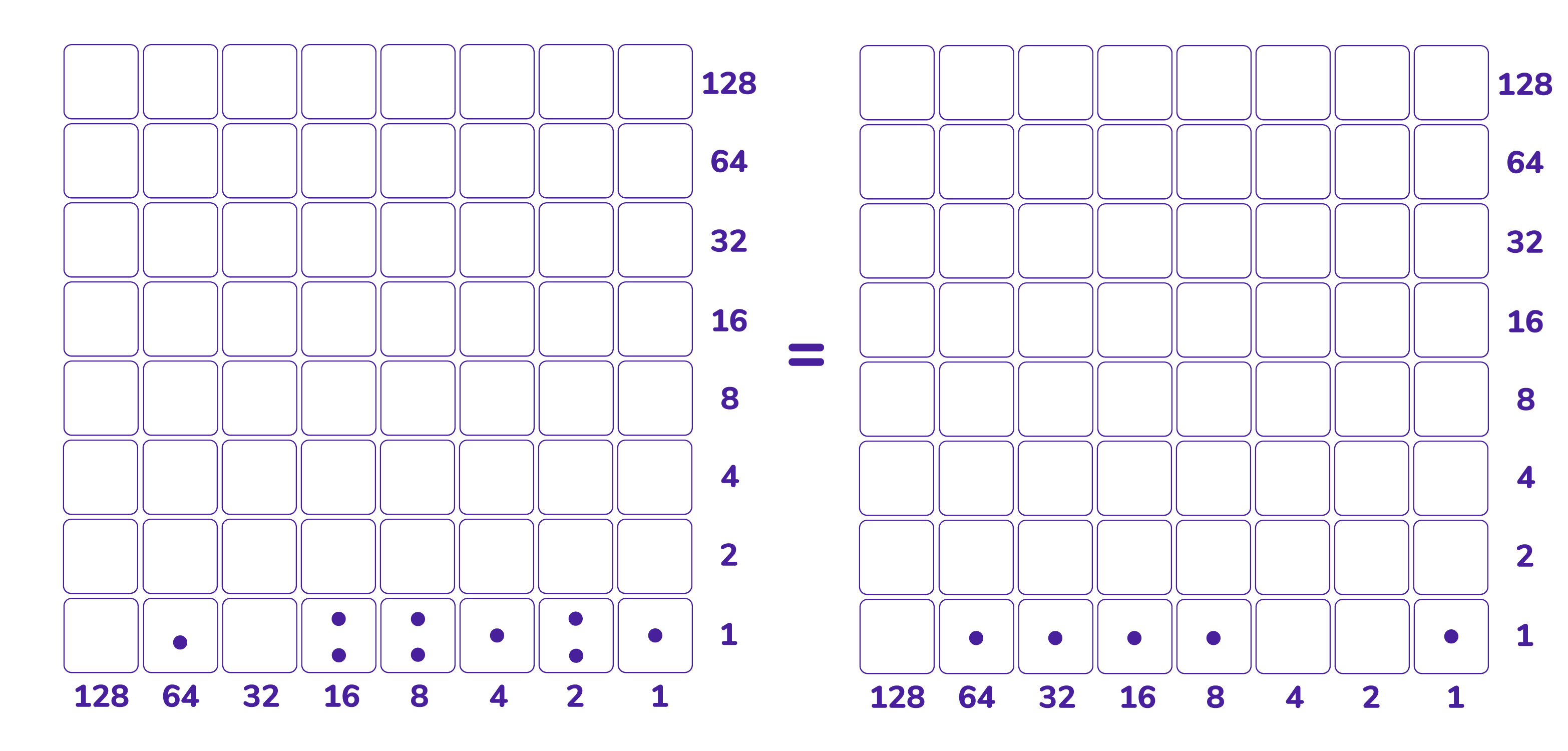Napier claimed that you can reverse this process and reconstruct the symmetric pattern of dots to see that $121$ is eleven squared.

a) Can you indeed slide the dots that represent $121$ on the bottom row diagonally upwards (or do some unexplosions and slide unexploded dots upwards) to recreate a picture of $11 \times 11$? The key is to focus on the northwest diagonal. Can you do this in a systematic way that you could explain your steps easily to a friend?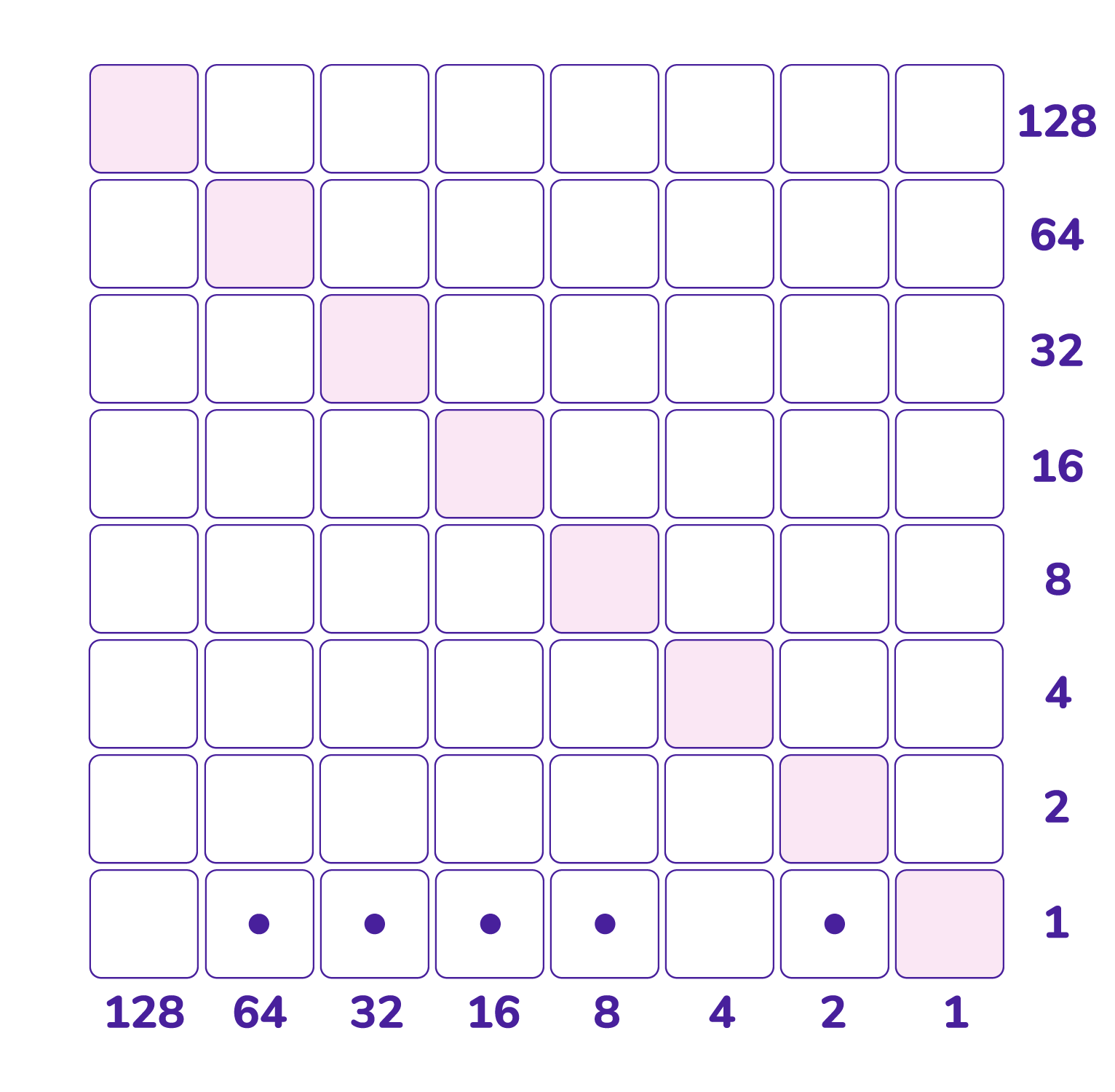b) Use your method with the number $145$ represented on the bottom row. Can you construct the picture of $12 \times 12$ (without knowing that one is looking for $12$ to begin with) along with one extra dot in the $1 \times 1$ cell?

c) Use Napier’s checkerboard to show that $1000 = 31^2+39$. (Again, presume you don’t know that you are looking for the number $31$.)

# EXPLORATION 2: NEGATIVE NUMBERS

In his book The Art of Computer Programming, Vol. 2. (1969) Donald Knuth introduces the negabinary system. Here every integer, positive and negative, is represented as a sum of powers of $-2$ using the coefficients $0$ and $1$.

In the language of Exploding Dots, negabinary is a $-1 \leftarrow 2$ machine where two dots in one box explode to be replaced by one antidot, one box to the left, and similarly two antidots in a box explode to be replaced by one dot, one box to the left.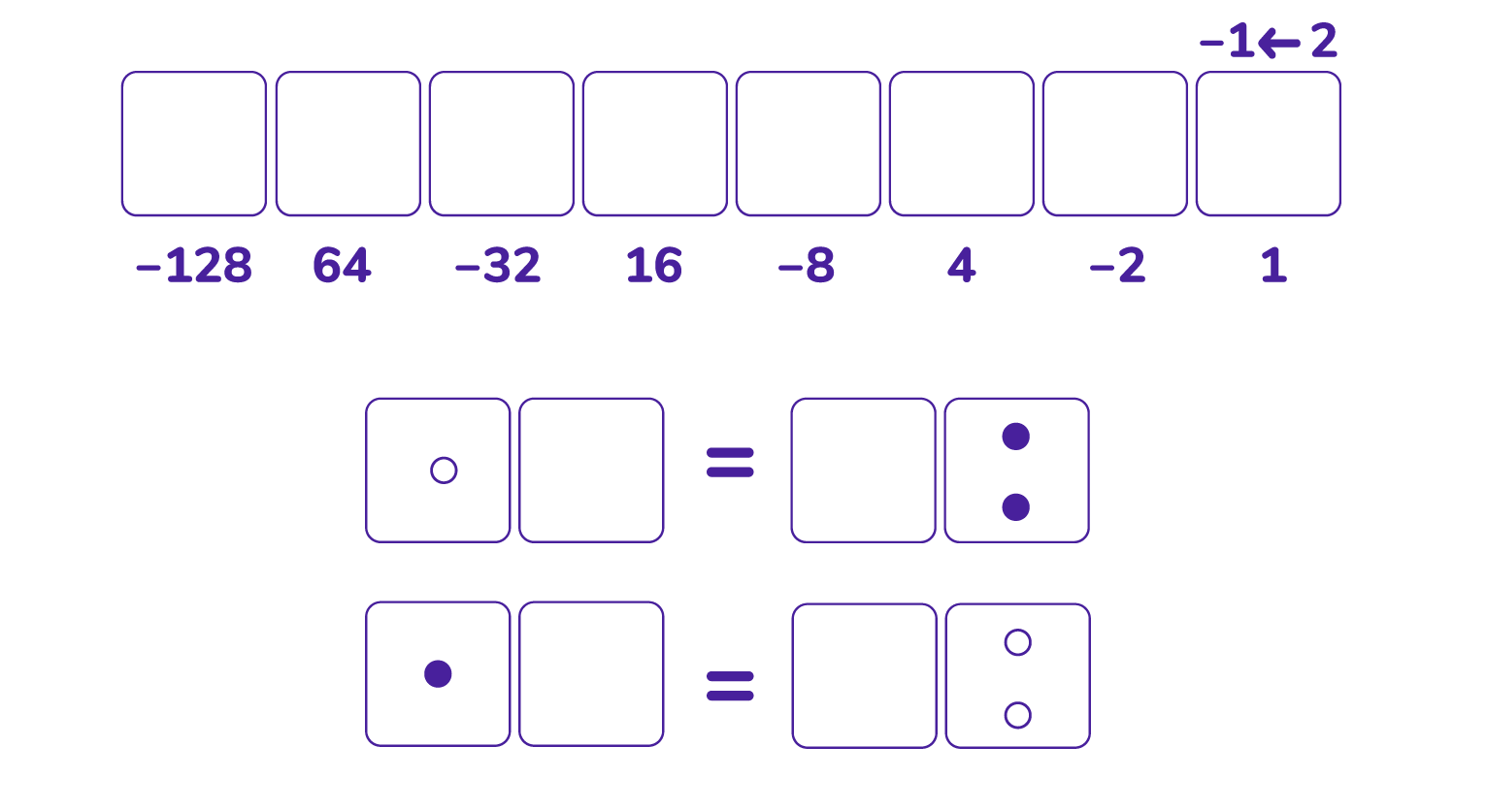But to avoid the appearance of antidots in the representations of numbers we observe that one antidot in a box is equivalent two dots, one in the original box and one, one place to the left.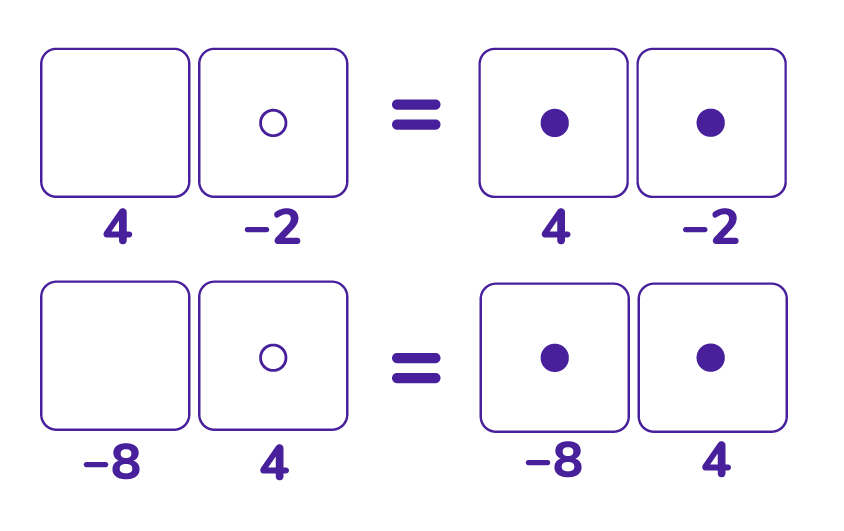Placing six dots in the $-1 \leftarrow 2$ machine and using this convention to avoid antidots gives the negabinary code $11010$ for six.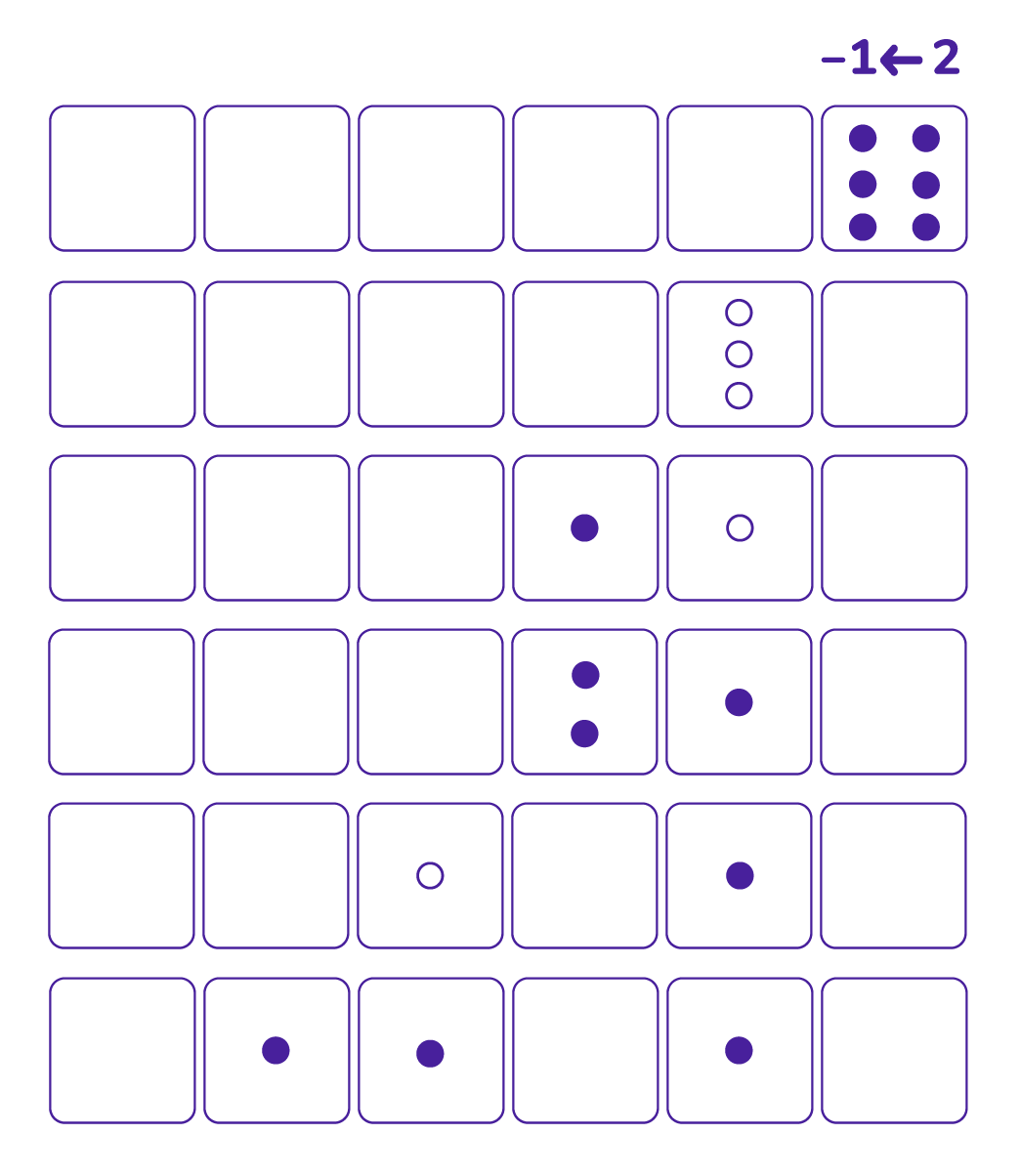The code for $-6$ in this machine is $1110$.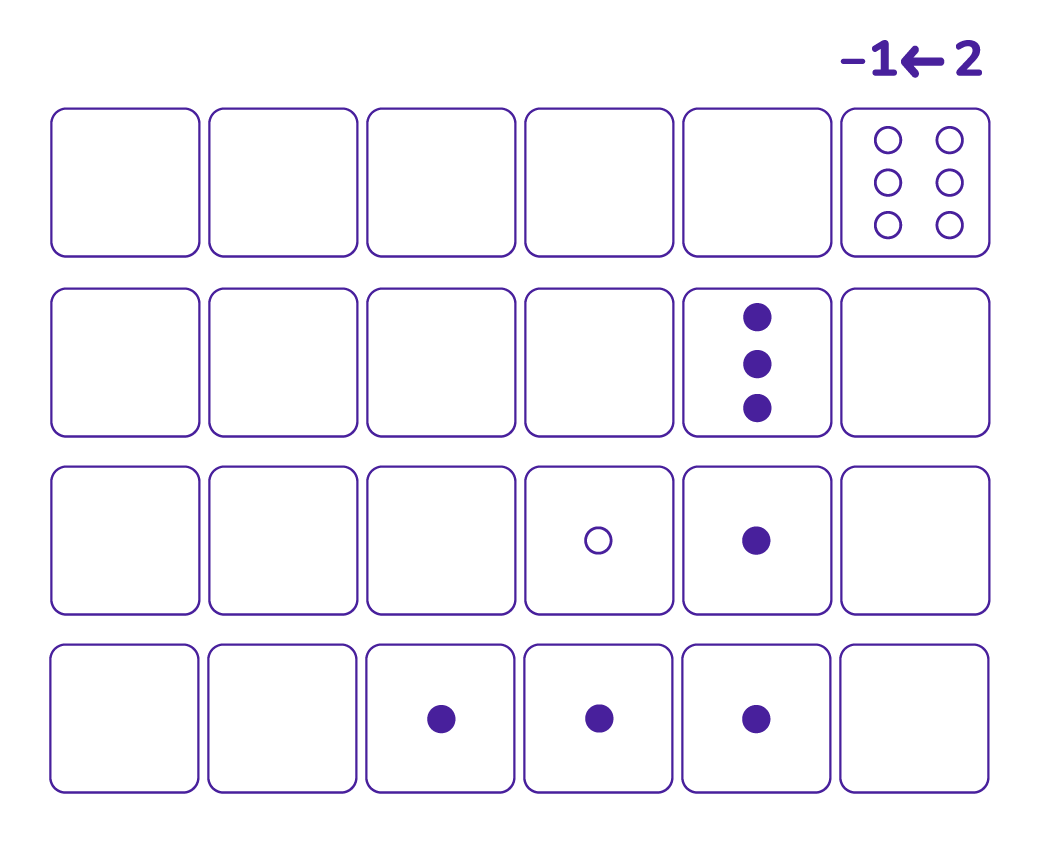a) Work out the negabinary codes of all the integers from $-10$ to $10$. Are there any patterns to be noticed and explained? (For example, which numbers give codes with an even number of digits? Which with an odd number of digits? Can you find a rule for divisibility by two? By three? Which numbers give palindromic codes?)

b) The $-1 \leftarrow 2$ machine shows that it is possible to represent each integer, positive or negative, in base $-2$ using only the digits $0$ and $1$ in at least one way. Prove that no integer can have two different base $-2$ representations using the digits $0$ and $1$.

Napier wasn’t using two differently colored counters in his work, one for dots and one for antidots. To follow suit, note that we can rephrase the rules of the $-1 \leftarrow 2$ machine solely in terms of dots.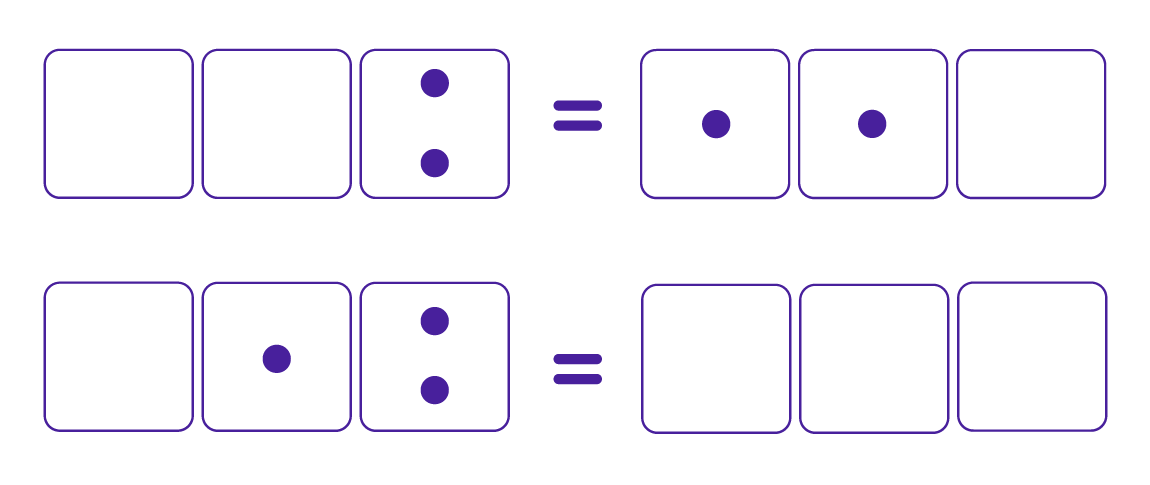Knuth suggests using Napier’s checkerboard with columns and rows labeled with values the powers of $-2$, representing numbers with counters in negabinary, and using the above two rules on the board (along with diagonal sliding) to manipulate pictures and thus do calculations.

For example, here is a picture of $6 \times \left(-6\right)$. Do you see how to obtain the answer $-36$ from it?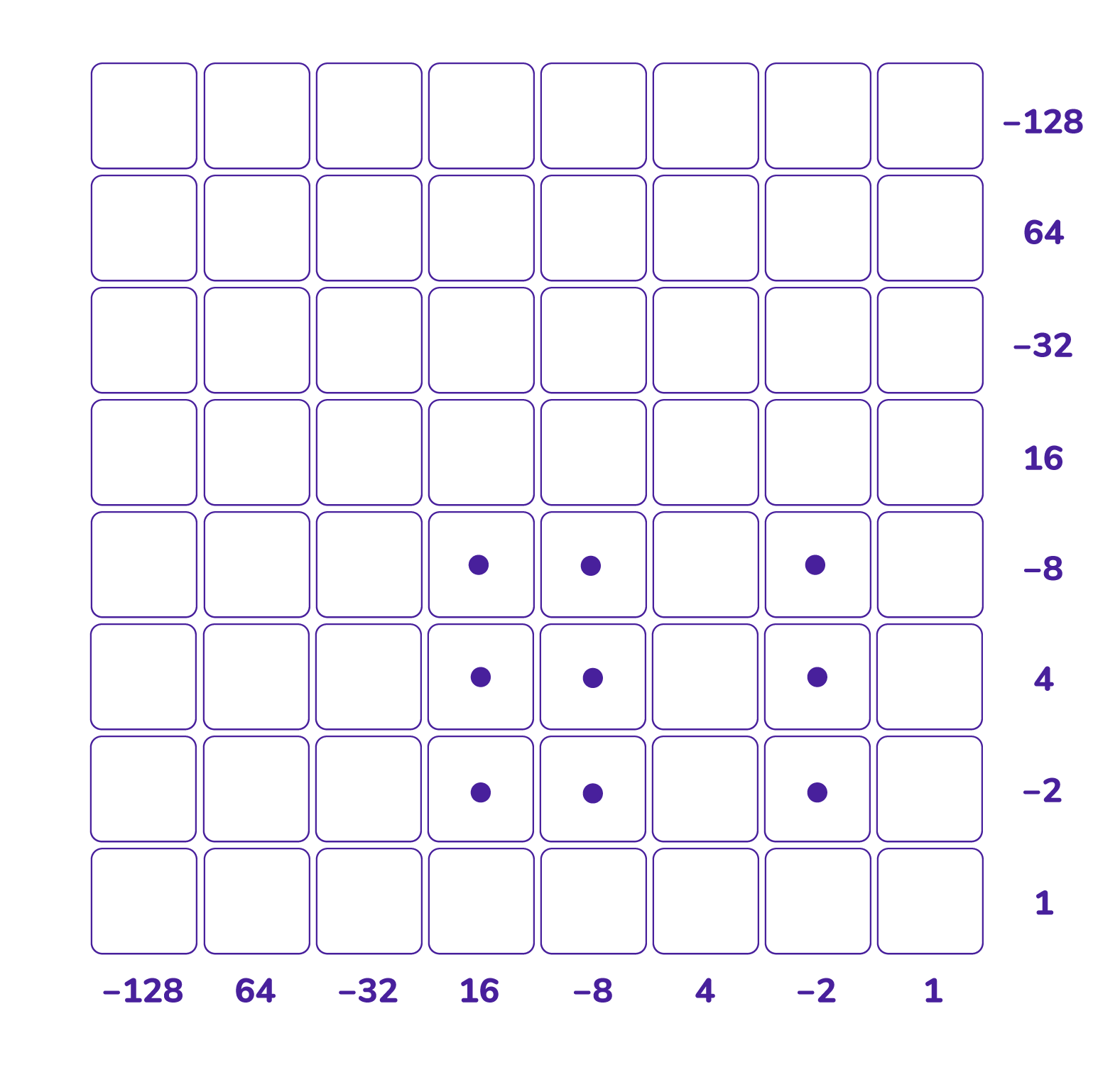c) Compute $6 + \left(-7\right)$ and $6 - \left(-7\right)$ and $6 \times \left(-7\right)$ in this negabinary checkerboard.

The number negative one has code $11$ in negabinary. So to change the sign of a number in negabinary we can multiply that number by $11$, that is, by $10+1$. Now multiplying a number by $1$ does not change the code of the number and multiplying by $10$ shifts all the digits of code one place to the left. So to change the sign of a number in negabinary we can write down the code for the number, write a same code with a zero addended, and add those two codes.

d) Compute $6 - \left(-7\right)$ as an addition problem of three terms: the code for $6$, the code for $-7$, and the code for $-7$ with a zero addended, all added together. Did you get the same answer as you did in part c)?

e) Is there a way to perform divisions on this board too? (Try $38 \div \left(-13\right)$.)